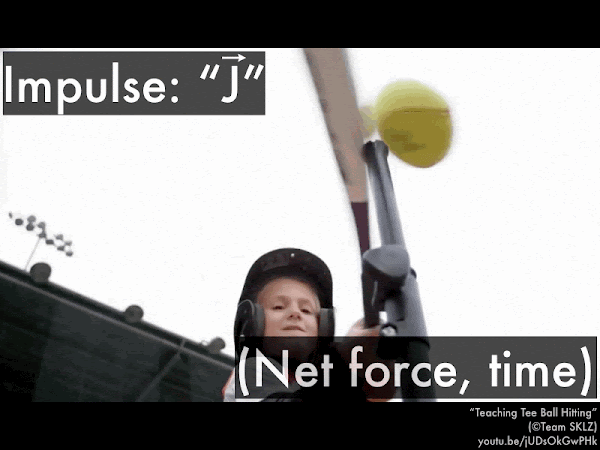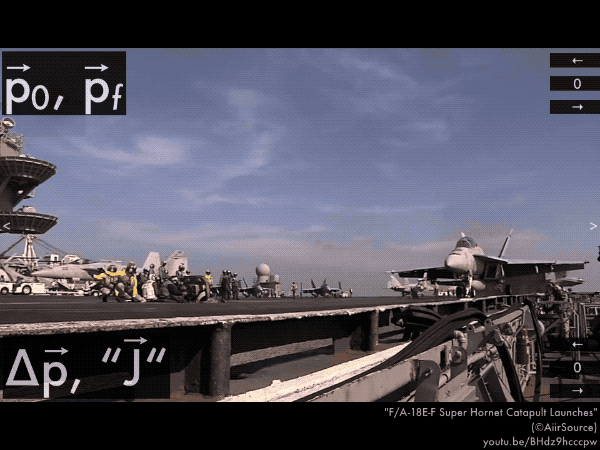## 20190930

### Online reading assignment: impulse and momentum

Physics 205A, fall semester 2019
Cuesta College, San Luis Obispo, CA

Students have a bi-weekly online reading assignment (hosted by SurveyMonkey.com), where they answer questions based on reading their textbook, material covered in previous lectures, opinion questions, and/or asking (anonymous) questions or making (anonymous) comments. Full credit is given for completing the online reading assignment before next week's lecture, regardless if whether their answers are correct/incorrect. Selected results/questions/comments are addressed by the instructor at the start of the following lecture.

The following questions were asked on reading textbook chapters and previewing a presentation on impulse and momentum.Selected/edited responses are given below.

Describe what you understand from the assigned textbook reading or presentation preview. Your description (2-3 sentences) should specifically demonstrate your level of understanding.
"I understand that to clearly determine momentum you must have mass to figure out the amount of force the object is projecting on to another object. Also, impulse is the change in momentum over time which is used to determine how much net force is applied to an object, or the momentum-impulse theory. Finally, impulse can change an object's direction from left-to-right (or vice versa)."

"Momentum takes into account mass and speed of an object. Impulse takes into account net force and duration of time. The impulse-momentum theorem reflects the order of effects."

"Impulse is related to average force times the change in time and linear momentum is equal to max times velocity."

"The definition of impulse seemed pretty straightforward and I feel like I understand that, but I honestly think I'm going to have trouble with this chapter. It didn't really make sense to me when I was reading it. I think I'm going to need more practice questions."

Describe what you found confusing from the assigned textbook reading or presentation preview. Your description (2-3 sentences) should specifically identify the concept(s) that you do not understand.
"How is the impulse-momentum theory useful? When do we need it?"

"I'm having trouble grasping the concept of the impulse-momentum theorem."

"This only has to do with when two objects are touching?"

"What I initially found confusing was the second example of the presentation preview and how the direction towards the left was negative. But understanding that the left direction was considered negative for all the examples, then it wasn't so confusing."

"I'm just having trouble visualizing how all this works for different situations."

"However, the confusing aspect of the textbook and presentation is going to be the examples using it. I feel like it might be difficult and get confusing with all the other equations."

"The setup of impulse-momentum theorem in relation to the examples given in the book, need some lecture and problems to work to make the connection to visual ideas."

"After going through the presentation preview I released impulse was slightly confusing. Once I read through it a second time I was able to understand it."

"This seems pretty straightforward."For the child hitting the tee ball with a bat, if the bat is swung such that it exerts the same net force on the tee ball for a longer time (by giving the bat more "follow-through"), the impulse on the tee ball will be __________, and the change in momentum of the tee ball will be:
 less; less. *****  less; greater. *******  greater; less. *****  greater; greater. ***************************  (Unsure/lost/guessing/help!) *For this golf ball initially at rest, and then has a speed of 97 m/s (to the right) after being hit by a golf club, indicate the horizontal directions (+/– signs) for the various impulse-momentum theorem quantities. (Only correct responses shown.)
Golf ball's initial momentum p0: no direction (0). [87%]
Golf ball's final momentum pf: to the right (+). [87%]
Golf ball's initial-to-final change in momentum ∆p: to the right (+). [89%]
Golf club's impulse "J" on the golf ball: to the right (+). [76%]For this F/A-18E-F Super Hornet initially at rest, and then has a speed of 74 m/s after being it is catapulted (to the left), indicate the horizontal directions (+/– signs) for these impulse-momentum theorem vectors. (Only correct responses shown.)
Super Hornet's initial momentum p0: no direction (0). [87%]
Super Hornet's final momentum pf: to the left (–). [76%]
Super Hornet's initial-to-final change in momentum ∆p: to the left (–). [71%]
Catapult's impulse "J" on the Super Hornet: to the left (–). [58%]For this Ford Ranger, hitting a crash barrier with a speed of 11.0 m/s (to the right), and then rebounding (to the left) off the crash barrier with a speed of 2.2 m/s, indicate the directions (+/– signs) for the various impulse-momentum theorem quantities. (Only correct responses shown.)
Ford Ranger's initial momentum p0: to the right (+). [87%]
Ford Ranger's final momentum pf: to the left (–). [78%]
Ford Ranger's initial-to-final change in momentum ∆p: to the left (–). [53%]
Crash barrier's impulse "J" on the Ford Ranger: to the left (–). [67%]
Ask the instructor an anonymous question, or make a comment. Selected questions/comments may be discussed in class.
"The impulse and the change in momentum is fun."

"I think I am not understanding the relationship between momentum and impulse."

"What actually is the impulse and how is it different than momentum?"

"I still don't understand the concept of finding the momentum or how the impulse is ever different than the change in momentum because I thought that was the same thing. I think I'm just lost." (Impulse causes the change in momentum, so calculating the impulse (caused by a force acting over a period of time) allows you to find out the change in momentum, since they're mathematically equal to each other.)

"Is it possible to have a change in momentum without an impulse?" (No. Since impulses cause changes in momentum, any change in an object's momentum (which has magnitude and direction) means that there must be an impulse acting on it. Also if there is no change in an object's momentum, the there is no impulse acting on it. (If those examples sound like Newton's second law and Newton's first law, then yes, they can be applied to the impulse-momentum theorem.)

"The concept behind 'impulse' is a little confusing. The name kind of implies that it's instantaneous and only happens at one point in time." (The common meaning of "impulse" means a sudden urge to act, but the older meaning comes from "impel," or to drive forward, urge, or command.)

"Is there a way to measure impulse in a lab setting, like with a special tool?" (Since impulse is the amount of force exerted over a duration in time, then all you would need to measure impulse is the force sensor to measure how much force is exerted, and a stopwatch to time how long you would exert that amount of force. But also since you have a motion sensor (or the video analysis tool) that can track the velocities of objects, you can use that to tell you the initial velocity and the final velocity of an object, and when you multiply those velocities with the object's mass, you can calculate the initial momentum and final momentum of the object; and the change in momentum (final momentum minus initial momentum) is also equal to the impulse.)

"Something I didn't understand is how is 'J' the impulse but it also mentions that 'F⋅Δt' is also impulse. There are many equations." (It's just a definition. You exert an impulse (denoted by the vector "J") on an object by exerting a force over a period of time.)

"Will these equations be given or do we have to memorize them?" (These impulse and momentum equations are given on the quizzes and exams.)

"I am very uncertain about my answers to these examples. I thought I understood the reading but I will definitely need some clarification of these examples in class please!"

"I hope I got these questions right."

"Please go over these in class! thx"

"This case of confusion only exists if I'm mistaken in my understanding of the relationship between impulse and momentum. Since impulse is the product of time and net force, the resulting change in momentum should also increase as is the tee ball case. If not, I'm lost and need help." (You should be okay, as your reasoning sounds good.)

"I'm a bit confused on the change in momentum example where a truck has an initial velocity of 11m/s to the right and bounces off of a crash barrier with a velocity of –2.2m/s to the left. I know that change in momentum is found by subtracting (∆pf – ∆p0). So, for this example, I'm assuming it would be m⋅((– 2.2m/s) – (+11m/s)) to find the direction of the truck's initial-to-final change in momentum ∆p, which would be mass times –13.2m/s to the left." (This looks good. Sounds like you aren't that confused at all.)

"When it comes to the energy transfer-balance equation, I'm struggling in knowing when to drop what is not needed and a little on how to set it up after." (If there is an energy form that doesn't apply, then you drop that term (for example, if there are no springs involved, then you can drop the ∆PEelas term. Or if there is no net initial-to-final change in that energy form, then you would also drop that term (for example, if an object is a rest on top of a vertical spring, which releases, and the object's final position is when it is (momentarily) at the highest point of its trajectory, then its initial and final translational kinetic energies are both zero, and then you would drop that term as ∆KEtrans = 0.)

"I'm enjoying this slightly cold weather."